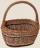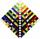# 6 terms

Find the first six terms of the sequence.

a1 = 7, an = an-1 + 6

Result

a1 =  7
a2 =  13
a3 =  19
a4 =  25
a5 =  31
a6 =  37

#### Solution:Leave us a comment of example and its solution (i.e. if it is still somewhat unclear...):

Showing 0 comments:Be the first to comment!## Next similar examples:

1. AP - simpleFind the first ten members of the sequence if a11 = 132, d = 3.
2. Nineteenth memberFind the nineteenth member of the arithmetic sequence: a1=33 d=5 find a19
3. AP - simpleDetermine the first nine elements of sequence if a10 = -1 and d = 4
4. Sequence 2Write the first 5 members of an arithmetic sequence a11=-14, d=-1
5. SequenceWrite the first 6 members of these sequence: a1 = 5 a2 = 7 an+2 = an+1 +2 an
6. SequenceWrite the first 7 members of an arithmetic sequence: a1=-3, d=6.
7. Sequence 3Write the first 5 members of an arithmetic sequence: a4=-35, a11=-105.
8. Functions f,gFind g(1) if g(x) = 3x - x2 Find f(5) if f(x) = x + 1/2
9. Apples in basketsDetermine how many apples are in baskets when in the first basket are 4 apples, and in any other is 29 apples more than the previous, and we have eight baskets.
10. SeatsSeats in the sport hall are organized so that each subsequent row has five more seats. First has 10 seats. How many seats are: a) in the eighth row b) in the eighteenth row
11. TreesA certain species of tree grows an average of 0.5 cm per week. Write an equation for the sequence that represents the weekly height of this tree in centimeters if the measurements begin when the tree is 200 centimeters tall.
12. AS sequenceIn an arithmetic sequence is given the difference d = -3 and a71 = 455. a) Determine the value of a62 b) Determine the sum of 71 members.
13. SequenceBetween numbers 1 and 53 insert n members of the arithmetic sequence that its sum is 702.
14. SeriesYour task is express the sum of the following arithmetic series for n = 14: S(n) = 11 + 13 + 15 + 17 + ... + 2n+9 + 2n+11
15. Linear systemSolve a set of two equations of two unknowns: 1.5x+1.2y=0.6 0.8x-0.2y=2
16. PowersExpress the expression ? as the n-th power of the base 10.
17. Theorem proveWe want to prove the sentense: If the natural number n is divisible by six, then n is divisible by three. From what assumption we started?